• 常数项时间（实现细节决定） 常数时间的操作 算数运算-加减乘除 位运算（ >>[带符号右移]/ >>>[不带符号右移]/ <</ |/ &/ ^） 赋值、比较、自增、子减 数组寻址 三种排序 选择排序：...
#algorithem/左神算法/基础 #sorting #XOR

评估算法优劣的核心标准

时间复杂度（流程决定）
额外空间复杂度（流程决定）
常数项时间（实现细节决定）

常数时间的操作

算数运算-加减乘除
位运算（ >>[带符号右移]/ >>>[不带符号右移]/ <</ |/ &/ ^）
赋值、比较、自增、子减
数组寻址

三种排序

选择排序：遍历整个数组找最大，放在最后
冒泡排序：相邻的两个数比较谁大谁放在后面
插入排序：第k次循环的时候，保证k角标之前的数组有序

第k个数字往前冒泡
::数据的初始状况会影响时间复杂度::

已排好的数组：O(N)
完全反着排的数组：O(N^2) -> 最差情况

对数器(用于测试)

有一个你想要测的方法a，
实现一个绝对正确但是复杂度不好的方法b
实现一个随机样本产生器
实现比对的方法
把方法a和方法b比对很多次来验证方法a是否正确。
如果有一个样本使得比对出错，打印样本分析是哪个方法出
错
当样本数量很多时比对测试依然正确，可以确定方法a已经
正确。

二分法：构建出一种可以排掉另外一边的逻辑

n * 2  + 1 = ( n << 1) | 1
eg. 局部最小数：返回一个无序的，去重的数组中 比左右的数都小的数

逻辑：通过判断中间的数和它相邻的数的大小关系来判断这两个数处于递增段还是递减段

	public static int getLessIndex(int[] arr) {
if (arr == null || arr.length == 0) {
return -1; // don't exists
}
if (arr.length == 1 || arr < arr) {
return 0; // arr号最小
}
if (arr[arr.length - 1] < arr[arr.length - 2]) {
return arr.length - 1; // arr[arr.length -1]号最小
}
// 最小值在中间
int left = 1;
int right = arr.length - 2;
int mid = 0;
while (left < right) {
mdi = (left + right) / 2;
if (arr[mid] > arr[mid - 1]) {
right = mid - 1;
} else if (arr[mid] > arr[mid + 1]) {
left = mid + 1;
} else {
return mid;
}
}
return left;
}


异或运算 ::无进位::相加

异或：相同为0，不同为1
同或：相同为1，不同为0
eg1. 不用额外变量交换两个数

int a = 3;
int b = 10;
a = a ^ b;
b = a ^ b;
a = a ^ b;

::注意：使用此方法必须保证要有两个空间，以下的例子为让arr变为零::
public static void main(String[] args) {
int[] arr = {7, 20 , 33};
// swap(arr, 0, 0); // 结果为{0, 20, 33}，因为交换需要两个空间，需要一个临时的空间来存储两个数的异或值
swap(arr, 0, 2);
}
public static void swap(int[] arr, int i, int j) {
arr[i] = arr[i] ^ arr[j];
arr[j] = arr[i] ^ arr[j];
arr[i] = arr[i] ^ arr[j];
}

- eg2. 一个数组中有一个数出现了奇数次，其他数都出现了偶数次，找到并打印这个数
- 直接对所有的数做异或运算
- eg3. 保留一个2进制数最右侧的1（找到这个1，其余为填充0）
- N & (~N + 1)
- eg4. 返回一个二进制数有多少位是1
- 根据eg3获取最右边的1, rightOne = N & (~N + 1)
- N ^ rightOne 抹掉最后一位, count++
- 循环上面两个步骤
- eg5. 一个数组中有2个数字(a, b)出现了奇数次，其余数字都出现偶数次，找到这两个数
- 第一次异或所有的数 -> eor = a ^ b
- a != b -> eor != 0 -> eor存在某些位上是1
- 任取值为1的一位[根据eg3我们能拿到最右侧的1]，在这一位上a != b [两种情况: a = 1, b = 0 或 a = 0, b = 1]
- 第二次异或所有这一位为1的数 -> eor’ -> eor’ 和eor ^ eor’即为a, b

public static void printOddTimesNum2(int[] arr) {
int eor = 0;
for (int i : arr) {
eor ^= i;
}
int rightOne = eor & (~eor + 1);
int eor2 = 0;
for ( int i : arr) {
if ((i & rightOne) != 0) { // 最里面的括号不能省略
eor2 ^= i;
}
}
System.out.println("第一个数为: " + eor2);
System.out.println("第二个数为: " + (eor2 ^ eor));
}



展开全文算法
• 2.评估算法指标：时间复杂度，额外空间复杂度，常数项时间复杂度 3.选择排序：从0到n-1位置找到最小值，跟0位置的数交换，从1到N-1位置上的再找到最小值，跟1位置交换，继续循环。。。 4.冒泡排序：从0开始一直...
1.在数据结构上玩流程，程序=数据结构+算法

2.评估算法指标：

时间复杂度；

额外空间复杂度；（与功能无关的空间）

常数项时间复杂度；

3.选择排序：从0到n-1位置找到最小值，跟0位置的数交换，从1到N-1位置上的再找到最小值，跟1位置交换，继续循环。。。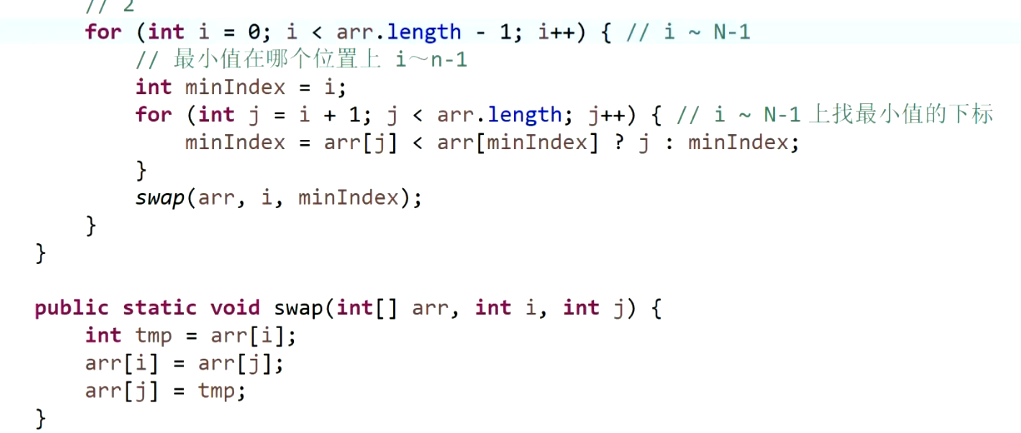4.冒泡排序：从0开始一直循环到N-1位置，从0开始，一个一个比较，比较0和1位置上谁大，大的交换到后面，遍历一次之后，最大的数，被放到了n-1位置上，继续从0开始遍历，第二次遍历到n-2位置上。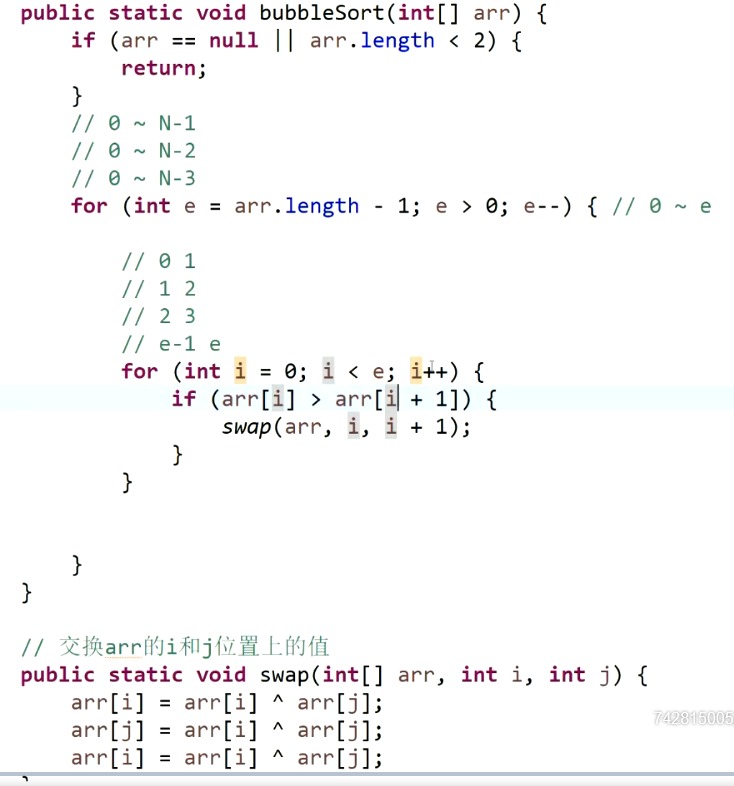5.冒泡排序和选择排序，性能不受数据的初始顺序影响，该做什么还是得做什么；

6.插入排序：先让0到0位置有序 ，第二步，让0到1位置有序，第三步，让0到2位置有序。。。如果比前一位小，一直往前换，直到0位置或者不比前一位小。数据的初始顺序会影响算法性能，如果数据直接有序，则不需要排序，最好时间复杂度O（n）,最坏时间复杂度O（n2）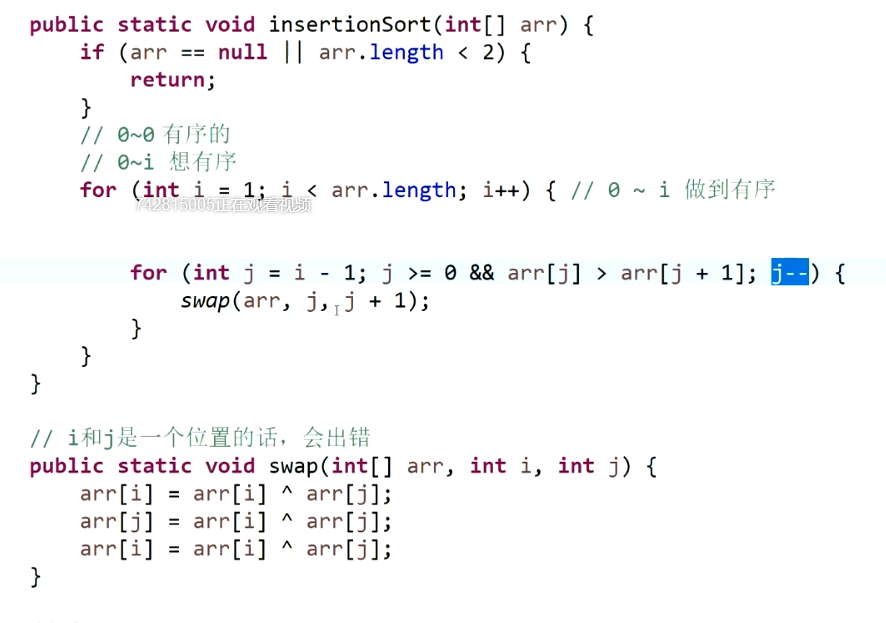7.确定算法流程的总操作数量和样本数量之间的关系表达式：

按照最差的情况估计；

把整个流程拆分为基本操作，保证每个基本操作都是常数时间复杂度；

如果数量为n,看看基本动作数量和n的关系；

O（n）就是代表最差的情况，最差时间复杂度；

8.最优解：

先满足时间复杂度，再满足空间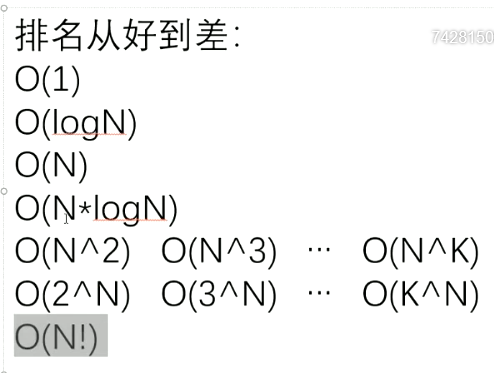9.算法分类：知道怎么算的算法；知道怎么试的算法

10.对数器：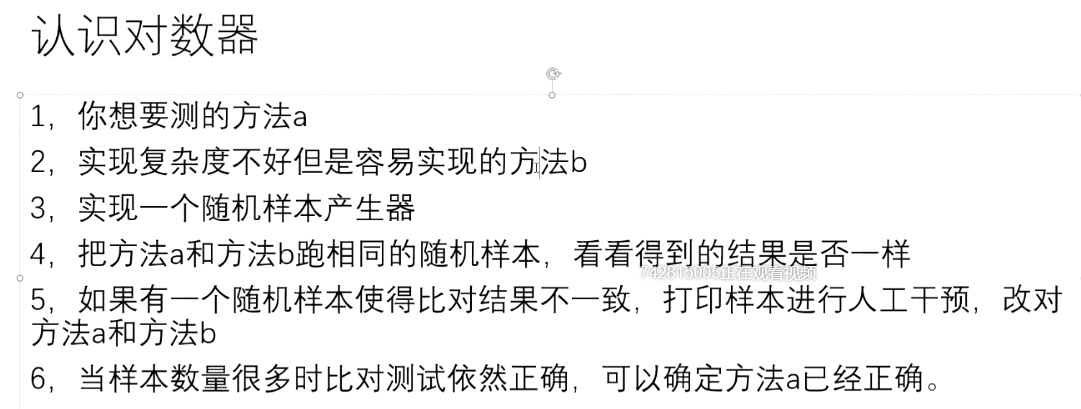11.二分法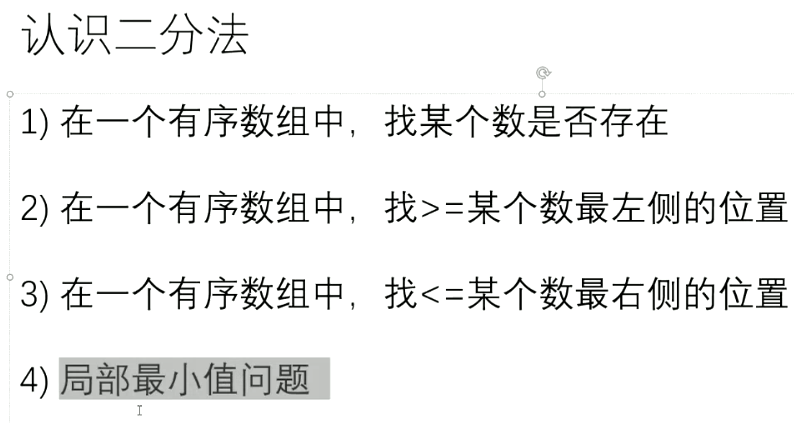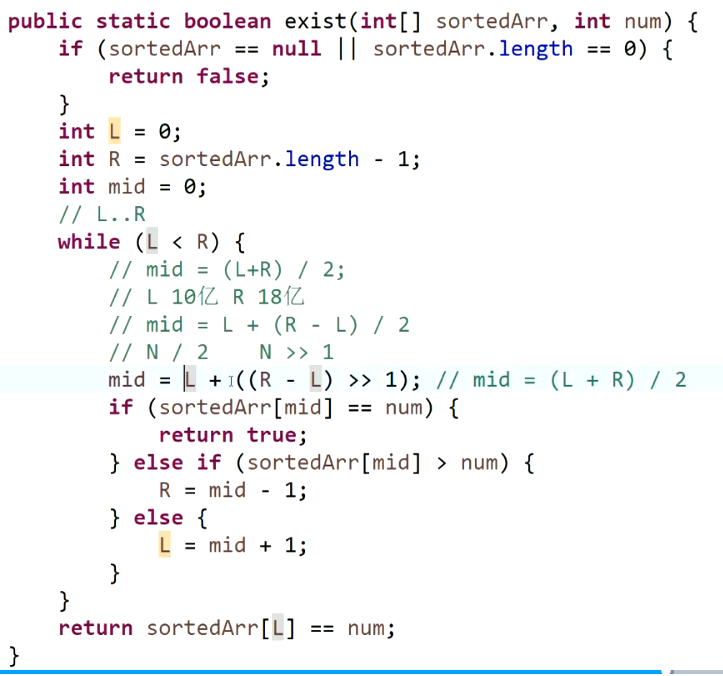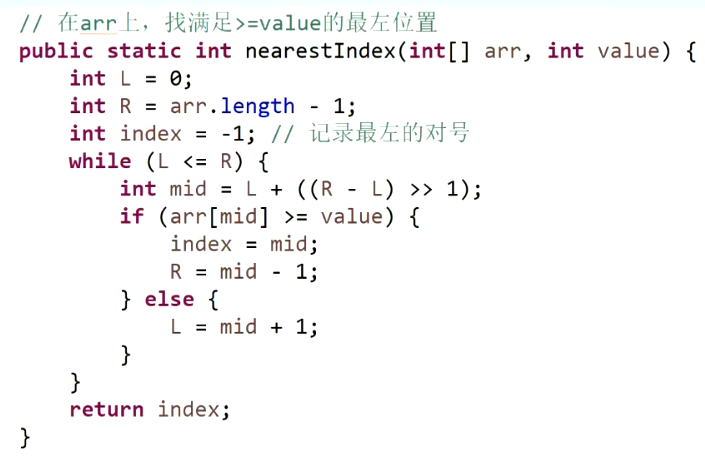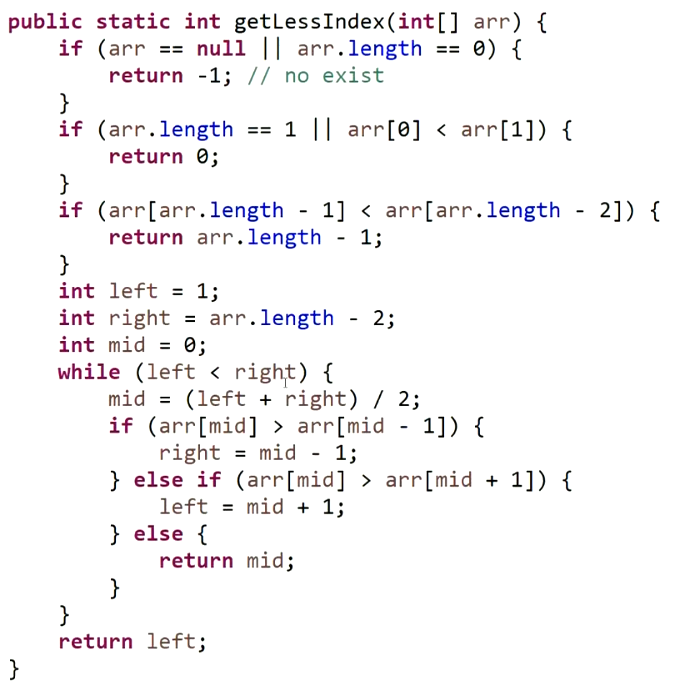12.认识异或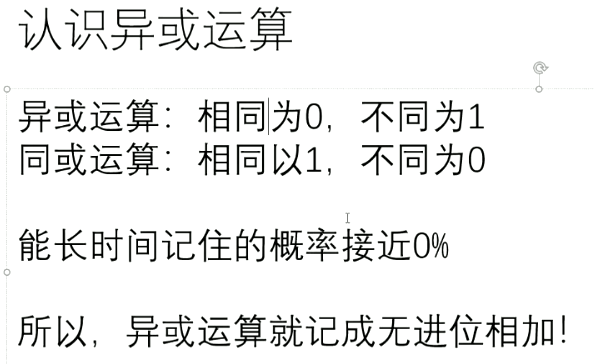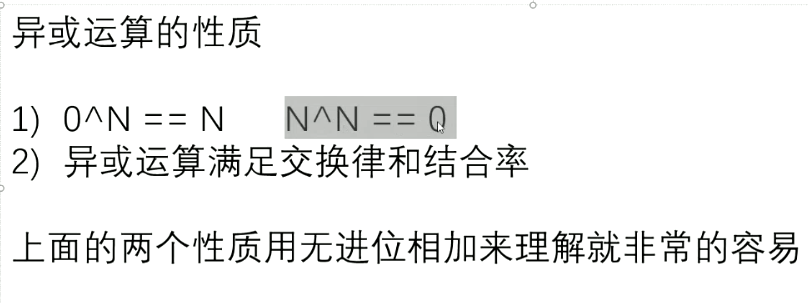不开辟额外空间交换两个值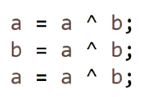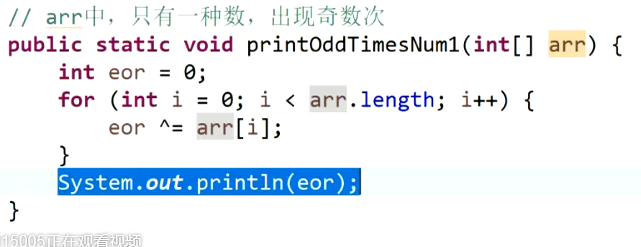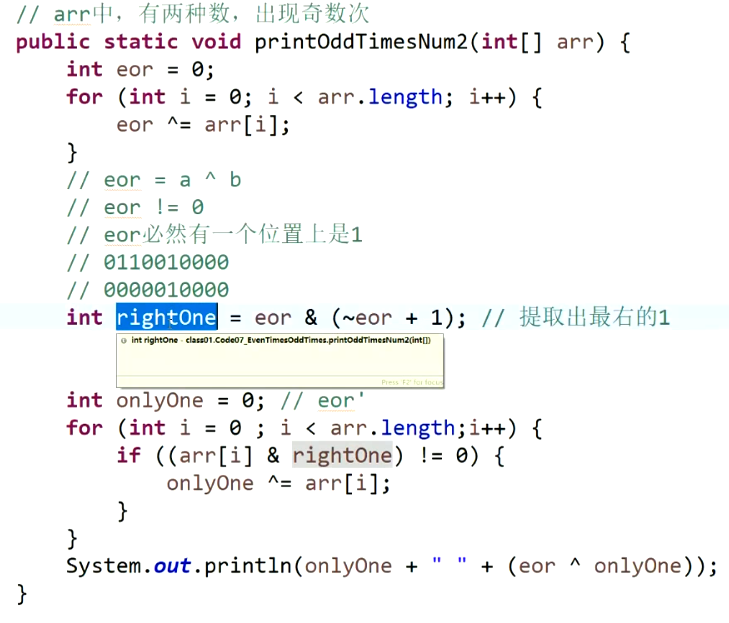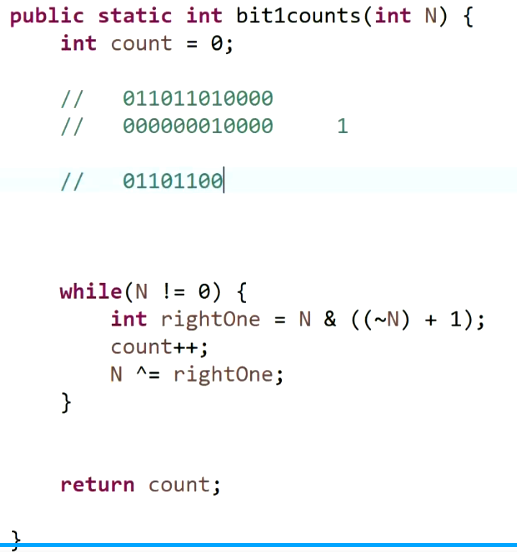展开全文• 1、四则运算 + - * / 、括弧()、正负(+ -) 2、百分数 %、求幂 ^ 、整数阶乘 ! (1 至 150) 3、参数符号计算，示例：a+b @@a=1,b=2 结算结果为3 用@@表示表达式中定义符号的值 4、常数e、圆周率PI 5、丰富的函数...
• 1、四则运算 + - * / 、括弧()、正负(+ -) 2、百分数 %、求幂 ^ 、整数阶乘 ! (1 至 150) 3、参数符号计算，示例：a+b @@a=1,b=2 结算结果为3 用@@表示表达式中定义符号的值 4、常数e、圆周率PI 5、丰富的函数...
• 文章目录1 时间复杂度、空间复杂度、排序、异或运算1.1 时间复杂度1.1.1 排序操作1.1.1.1 选择排序1.1.1.2 冒泡排序1.1.1.3 插入排序1.2 空间复杂度1.3 常数项时间复杂度1.4 算法最优解1.5 常见时间复杂度1.6 算法和...
文章目录1 时间复杂度、空间复杂度、排序、异或运算1.1 时间复杂度1.1.1 排序操作1.1.1.1 选择排序1.1.1.2 冒泡排序1.1.1.3 插入排序1.2 空间复杂度1.3 常数项时间复杂度1.4 算法最优解1.5 常见时间复杂度1.6 算法和数据结构脉络1.7 认识对数器1.8 认识二分法1.9 认识异或运算
1 时间复杂度、空间复杂度、排序、异或运算
1.1 时间复杂度

常数时间操作：

算数运算：+ - * /
位运算：>>（带符号右移动）、 >>>（不带符号右移动） 、 <<、 | 、& 、^

带符号就是最高位补符号位，不带符号就是最高位补0

赋值操作：比较，自增，自减操作
数组寻址等

总之，执行时间固定的操作都是常数时间的操作。反之执行时间不固定的操作，都不是常数时间的操作

通过基本动作的常数时间，推导时间复杂度

对于双层循环来说，n*（常数）+ (n-1)(常数)+ … + 2(常数) + 1*（常数） => 推导出

y = an^2 + bn + c

忽略掉低阶项，忽略掉常数项，忽略掉高阶项的系数，得到时间复杂度为n^2
1.1.1 排序操作
1.1.1.1 选择排序
package class01;

import java.util.Arrays;

public class Code01_SelectionSort {

public static void selectionSort(int[] arr) {
if (arr == null || arr.length < 2) {
return;
}
// 0 ~ N-1
// 1~n-1
// 2
for (int i = 0; i < arr.length - 1; i++) { // i ~ N-1
// 最小值在哪个位置上  i～n-1
int minIndex = i;
for (int j = i + 1; j < arr.length; j++) { // i ~ N-1 上找最小值的下标
minIndex = arr[j] < arr[minIndex] ? j : minIndex;
}
swap(arr, i, minIndex);
}
}

public static void swap(int[] arr, int i, int j) {
int tmp = arr[i];
arr[i] = arr[j];
arr[j] = tmp;
}

// for test
public static void comparator(int[] arr) {
Arrays.sort(arr);
}

// for test
public static int[] generateRandomArray(int maxSize, int maxValue) {
// Math.random()   [0,1)
// Math.random() * N  [0,N)
// (int)(Math.random() * N)  [0, N-1]
int[] arr = new int[(int) ((maxSize + 1) * Math.random())];
for (int i = 0; i < arr.length; i++) {
// [-? , +?]
arr[i] = (int) ((maxValue + 1) * Math.random()) - (int) (maxValue * Math.random());
}
return arr;
}

// for test
public static int[] copyArray(int[] arr) {
if (arr == null) {
return null;
}
int[] res = new int[arr.length];
for (int i = 0; i < arr.length; i++) {
res[i] = arr[i];
}
return res;
}

// for test 对数器
public static boolean isEqual(int[] arr1, int[] arr2) {
if ((arr1 == null && arr2 != null) || (arr1 != null && arr2 == null)) {
return false;
}
if (arr1 == null && arr2 == null) {
return true;
}
if (arr1.length != arr2.length) {
return false;
}
for (int i = 0; i < arr1.length; i++) {
if (arr1[i] != arr2[i]) {
return false;
}
}
return true;
}

// for test
public static void printArray(int[] arr) {
if (arr == null) {
return;
}
for (int i = 0; i < arr.length; i++) {
System.out.print(arr[i] + " ");
}
System.out.println();
}

// for test
public static void main(String[] args) {
int testTime = 500000;
int maxSize = 100;
int maxValue = 100;
boolean succeed = true;
for (int i = 0; i < testTime; i++) {
int[] arr1 = generateRandomArray(maxSize, maxValue);
int[] arr2 = copyArray(arr1);
selectionSort(arr1);
comparator(arr2);
if (!isEqual(arr1, arr2)) {
succeed = false;
printArray(arr1);
printArray(arr2);
break;
}
}
System.out.println(succeed ? "Nice!" : "Fucking fucked!");

int[] arr = generateRandomArray(maxSize, maxValue);
printArray(arr);
selectionSort(arr);
printArray(arr);
}

}

1.1.1.2 冒泡排序
package class01;

import java.util.Arrays;

public class Code02_BubbleSort {

public static void bubbleSort(int[] arr) {
if (arr == null || arr.length < 2) {
return;
}
// 0 ~ N-1
// 0 ~ N-2
// 0 ~ N-3
for (int e = arr.length - 1; e > 0; e--) { // 0 ~ e
for (int i = 0; i < e; i++) {
if (arr[i] > arr[i + 1]) {
swap(arr, i, i + 1);
}
}
}
}

// 交换arr的i和j位置上的值
public static void swap(int[] arr, int i, int j) {
arr[i] = arr[i] ^ arr[j];
arr[j] = arr[i] ^ arr[j];
arr[i] = arr[i] ^ arr[j];
}

// for test
public static void comparator(int[] arr) {
Arrays.sort(arr);
}

// for test
public static int[] generateRandomArray(int maxSize, int maxValue) {
int[] arr = new int[(int) ((maxSize + 1) * Math.random())];
for (int i = 0; i < arr.length; i++) {
arr[i] = (int) ((maxValue + 1) * Math.random()) - (int) (maxValue * Math.random());
}
return arr;
}

// for test
public static int[] copyArray(int[] arr) {
if (arr == null) {
return null;
}
int[] res = new int[arr.length];
for (int i = 0; i < arr.length; i++) {
res[i] = arr[i];
}
return res;
}

// for test
public static boolean isEqual(int[] arr1, int[] arr2) {
if ((arr1 == null && arr2 != null) || (arr1 != null && arr2 == null)) {
return false;
}
if (arr1 == null && arr2 == null) {
return true;
}
if (arr1.length != arr2.length) {
return false;
}
for (int i = 0; i < arr1.length; i++) {
if (arr1[i] != arr2[i]) {
return false;
}
}
return true;
}

// for test
public static void printArray(int[] arr) {
if (arr == null) {
return;
}
for (int i = 0; i < arr.length; i++) {
System.out.print(arr[i] + " ");
}
System.out.println();
}

// for test
public static void main(String[] args) {
int testTime = 500000;
int maxSize = 100;
int maxValue = 100;
boolean succeed = true;
for (int i = 0; i < testTime; i++) {
int[] arr1 = generateRandomArray(maxSize, maxValue);
int[] arr2 = copyArray(arr1);
bubbleSort(arr1);
comparator(arr2);
if (!isEqual(arr1, arr2)) {
succeed = false;
break;
}
}
System.out.println(succeed ? "Nice!" : "Fucking fucked!");

int[] arr = generateRandomArray(maxSize, maxValue);
printArray(arr);
bubbleSort(arr);
printArray(arr);
}

}

1.1.1.3 插入排序
package class01;

import java.util.Arrays;

public class Code03_InsertionSort {

public static void insertionSort(int[] arr) {
if (arr == null || arr.length < 2) {
return;
}
// 0~0 有序的
// 0~i 想有序
for (int i = 1; i < arr.length; i++) { // 0 ~ i 做到有序
for (int j = i - 1; j >= 0 && arr[j] > arr[j + 1]; j--) {
swap(arr, j, j + 1);
}
}
}

// i和j是一个位置的话，会出错
public static void swap(int[] arr, int i, int j) {
arr[i] = arr[i] ^ arr[j];
arr[j] = arr[i] ^ arr[j];
arr[i] = arr[i] ^ arr[j];
}

// for test
public static void comparator(int[] arr) {
Arrays.sort(arr);
}

// for test
public static int[] generateRandomArray(int maxSize, int maxValue) {
// Math.random() ->  [0,1) 所有的小数，等概率返回一个
// Math.random() * N -> [0,N) 所有小数，等概率返回一个
// (int)(Math.random() * N) -> [0,N-1] 所有的整数，等概率返回一个
int[] arr = new int[(int) ((maxSize + 1) * Math.random())]; // 长度随机
for (int i = 0; i < arr.length; i++) {
arr[i] = (int) ((maxValue + 1) * Math.random())
- (int) (maxValue * Math.random());
}
return arr;
}

// for test
public static int[] copyArray(int[] arr) {
if (arr == null) {
return null;
}
int[] res = new int[arr.length];
for (int i = 0; i < arr.length; i++) {
res[i] = arr[i];
}
return res;
}

// for test
public static boolean isEqual(int[] arr1, int[] arr2) {
if ((arr1 == null && arr2 != null) || (arr1 != null && arr2 == null)) {
return false;
}
if (arr1 == null && arr2 == null) {
return true;
}
if (arr1.length != arr2.length) {
return false;
}
for (int i = 0; i < arr1.length; i++) {
if (arr1[i] != arr2[i]) {
return false;
}
}
return true;
}

// for test
public static void printArray(int[] arr) {
if (arr == null) {
return;
}
for (int i = 0; i < arr.length; i++) {
System.out.print(arr[i] + " ");
}
System.out.println();
}

// for test
public static void main(String[] args) {
int testTime = 500000;
int maxSize = 100; // 随机数组的长度0～100
int maxValue = 100;// 值：-100～100
boolean succeed = true;
for (int i = 0; i < testTime; i++) {
int[] arr1 = generateRandomArray(maxSize, maxValue);
int[] arr2 = copyArray(arr1);
insertionSort(arr1);
comparator(arr2);
if (!isEqual(arr1, arr2)) {
// 打印arr1
// 打印arr2
succeed = false;
break;
}
}
System.out.println(succeed ? "Nice!" : "Fucking fucked!");

int[] arr = generateRandomArray(maxSize, maxValue);
printArray(arr);
insertionSort(arr);
printArray(arr);
}

}


插入排序和前面两种排序的不同是在于，插入排序跟数组初始顺序有关，在初始有序的情况下，有可能时间复杂度为O(N),有可能为O(N ^2)，但是我们估计时间复杂度要按照最差的情况来估计，所以插入排序的时间复杂度仍然O(N ^2)
1.2 空间复杂度
申请有限几个变量，和样本量n没关系，就是空间复杂度O(1)，如果要开辟一个空间数组和样本量n是一样大，用来支持我们的算法流程那么O(N)。反之用户就是要实现数组拷贝，我们开辟一个新的n大小数组用来支撑用户的需求，那么仍然是O(1)
1.3 常数项时间复杂度
如果两个相同时间复杂度的算法要比较性能，这个时候需要比较单个常数项时间，对能力要求较高，没有意义，不如样本量试验实际测试来比较
1.4 算法最优解
我们认为最优解的考虑顺序是，先满足时间复杂度指标，再去使用较少的空间。一般来说，算法题，ACM等不会卡常数项时间
1.5 常见时间复杂度

依次从好到坏 O(1) -> O(logN) -> O(N) -> O(N*logN) -> O(N^2) -> O(N^3) … -> O(N!)

1.6 算法和数据结构脉络

知道怎么算的算法
知道怎么试的算法(递归)

1.7 认识对数器

准备你想要测试的方法a
实现一个复杂度不好，但是容易实现的方法b
实现一个随机样本产生器
把方法a和方法b跑相同的随机样本，看看得到的结果是否一样
如果有一个随机样本使得对比结果不一致，打印样本进行人工干预，改对方法a和方法b
当样本数量很多，测试对比依然正确，可以确定方法a已经正确

1.8 认识二分法

在一个有序数组中，找某个数是否存在

二分查找值，基于有序数组，算法复杂度为二分了多少次，O(log2N)可以写成O(logN)

123579

package class01;

import java.util.Arrays;

public class Code04_BSExist {

public static boolean exist(int[] sortedArr, int num) {
if (sortedArr == null || sortedArr.length == 0) {
return false;
}
int L = 0;
int R = sortedArr.length - 1;
int mid = 0;
// L..R
while (L < R) {
// mid = (L+R) / 2;
// L 10亿  R 18亿
// mid = L + (R - L) / 2
// N / 2    N >> 1
mid = L + ((R - L) >> 1); // mid = (L + R) / 2
if (sortedArr[mid] == num) {
return true;
} else if (sortedArr[mid] > num) {
R = mid - 1;
} else {
L = mid + 1;
}
}
return sortedArr[L] == num;
}

// for test
public static boolean test(int[] sortedArr, int num) {
for(int cur : sortedArr) {
if(cur == num) {
return true;
}
}
return false;
}

// for test
public static int[] generateRandomArray(int maxSize, int maxValue) {
int[] arr = new int[(int) ((maxSize + 1) * Math.random())];
for (int i = 0; i < arr.length; i++) {
arr[i] = (int) ((maxValue + 1) * Math.random()) - (int) (maxValue * Math.random());
}
return arr;
}

public static void main(String[] args) {
int testTime = 500000;
int maxSize = 10;
int maxValue = 100;
boolean succeed = true;
for (int i = 0; i < testTime; i++) {
int[] arr = generateRandomArray(maxSize, maxValue);
Arrays.sort(arr);
int value = (int) ((maxValue + 1) * Math.random()) - (int) (maxValue * Math.random());
if (test(arr, value) != exist(arr, value)) {
succeed = false;
break;
}
}
System.out.println(succeed ? "Nice!" : "Fucking fucked!");
}

}


在一个有序数组中，找>=某个数最左侧的位置

122222333578888999999 找大于等于2最左侧的位置

package class01;

import java.util.Arrays;

public class Code05_BSNearLeft {

// 在arr上，找满足>=value的最左位置
public static int nearestIndex(int[] arr, int value) {
int L = 0;
int R = arr.length - 1;
int index = -1; // 记录最左的对号
while (L <= R) {
int mid = L + ((R - L) >> 1);
if (arr[mid] >= value) {
index = mid;
R = mid - 1;
} else {
L = mid + 1;
}
}
return index;
}

// for test
public static int test(int[] arr, int value) {
for (int i = 0; i < arr.length; i++) {
if (arr[i] >= value) {
return i;
}
}
return -1;
}

// for test
public static int[] generateRandomArray(int maxSize, int maxValue) {
int[] arr = new int[(int) ((maxSize + 1) * Math.random())];
for (int i = 0; i < arr.length; i++) {
arr[i] = (int) ((maxValue + 1) * Math.random()) - (int) (maxValue * Math.random());
}
return arr;
}

// for test
public static void printArray(int[] arr) {
if (arr == null) {
return;
}
for (int i = 0; i < arr.length; i++) {
System.out.print(arr[i] + " ");
}
System.out.println();
}

public static void main(String[] args) {
int testTime = 500000;
int maxSize = 10;
int maxValue = 100;
boolean succeed = true;
for (int i = 0; i < testTime; i++) {
int[] arr = generateRandomArray(maxSize, maxValue);
Arrays.sort(arr);
int value = (int) ((maxValue + 1) * Math.random()) - (int) (maxValue * Math.random());
if (test(arr, value) != nearestIndex(arr, value)) {
printArray(arr);
System.out.println(value);
System.out.println(test(arr, value));
System.out.println(nearestIndex(arr, value));
succeed = false;
break;
}
}
System.out.println(succeed ? "Nice!" : "Fucking fucked!");
}

}


在一个有序数组中，找<=某个数最右侧的位置


package class01;

import java.util.Arrays;

public class Code05_BSNearRight {

// 在arr上，找满足<=value的最右位置
public static int nearestIndex(int[] arr, int value) {
int L = 0;
int R = arr.length - 1;
int index = -1; // 记录最右的对号
while (L <= R) {
int mid = L + ((R - L) >> 1);
if (arr[mid] <= value) {
index = mid;
L = mid + 1;
} else {
R = mid - 1;
}
}
return index;
}

// for test
public static int test(int[] arr, int value) {
for (int i = arr.length - 1; i >= 0; i--) {
if (arr[i] <= value) {
return i;
}
}
return -1;
}

// for test
public static int[] generateRandomArray(int maxSize, int maxValue) {
int[] arr = new int[(int) ((maxSize + 1) * Math.random())];
for (int i = 0; i < arr.length; i++) {
arr[i] = (int) ((maxValue + 1) * Math.random()) - (int) (maxValue * Math.random());
}
return arr;
}

// for test
public static void printArray(int[] arr) {
if (arr == null) {
return;
}
for (int i = 0; i < arr.length; i++) {
System.out.print(arr[i] + " ");
}
System.out.println();
}

public static void main(String[] args) {
int testTime = 500000;
int maxSize = 10;
int maxValue = 100;
boolean succeed = true;
for (int i = 0; i < testTime; i++) {
int[] arr = generateRandomArray(maxSize, maxValue);
Arrays.sort(arr);
int value = (int) ((maxValue + 1) * Math.random()) - (int) (maxValue * Math.random());
if (test(arr, value) != nearestIndex(arr, value)) {
printArray(arr);
System.out.println(value);
System.out.println(test(arr, value));
System.out.println(nearestIndex(arr, value));
succeed = false;
break;
}
}
System.out.println(succeed ? "Nice!" : "Fucking fucked!");
}

}



局部最小值问题

无序数组，任意两个相邻的数不相等，返回一个局部最小值

package class01;

public class Code06_BSAwesome {

public static int getLessIndex(int[] arr) {
if (arr == null || arr.length == 0) {
return -1; // no exist
}
if (arr.length == 1 || arr < arr) {
return 0;
}
if (arr[arr.length - 1] < arr[arr.length - 2]) {
return arr.length - 1;
}
int left = 1;
int right = arr.length - 2;
int mid = 0;
while (left < right) {
mid = (left + right) / 2;
if (arr[mid] > arr[mid - 1]) {
right = mid - 1;
} else if (arr[mid] > arr[mid + 1]) {
left = mid + 1;
} else {
return mid;
}
}
return left;
}

}

1.9 认识异或运算
异或运算：相同为0，不同为1
同或运算：相同为1， 不同为0，不掌握
上述特别不容易记住，异或运算就记成无进位相加：比如十进制6异或7，就理解为110和111按位不进位相加,得到001

所以 0^N = N ，  N^N = 0
异或运算满足交换律和结合律,所以A异或B异或C = A异或(B异或C) = (A异或C)异或B

题目一：如何不用额外变量就交换两个数
a = x b = y两个数交换位置

a = a ^ b # 第一步操作，此时 a = x^y , b=y
b = a ^ b # 第二步操作，此时 a = x^y , b = x^y^y => b = x^0 => b = x
a = a ^ b # 第三步操作，此时 a = x^y^x, b = x, a=> x^x^y => a=y

三步操作，实现交换ab的值


package class01;

public class Test {

public static void main(String[] args) {
int a = 6;
int b = 6;

a = a ^ b;
b = a ^ b;
a = a ^ b;

System.out.println(a);
System.out.println(b);

int[] arr = {3,1,100};

System.out.println(arr);
System.out.println(arr);

swap(arr, 0, 0);

System.out.println(arr);
System.out.println(arr);

}

public static void swap (int[] arr, int i, int j) {
// arr = arr ^ arr;
arr[i]  = arr[i] ^ arr[j];
arr[j]  = arr[i] ^ arr[j];
arr[i]  = arr[i] ^ arr[j];
}

}

注意，如果a和b指向同一块内存，改方法不可行
题目二：一个数组中有一种数出现了奇数次，其他数都出现了偶数次，怎么找到并打印这种数

[2,2,1,3,2,3,2,1,1] 数组中存在四个2，两个3，三个1，定义一个常量等于0，分别对该数组中的数遍历一遍进行异或，最后，该变量等于多少，那么奇数的值就是多少。因为异或运算满足交换和结合律

题目三：怎么把一个int类型的数，提取出最右侧的1来

n与上（n取反加1）即可 => N & ( (~N)+1 )

题目四：一个数组中有两种不相等的数出现了奇数次，其他数出现了偶数次，怎么找到并打印这两种数

定义一个常量eor = 0，分别对该数组每个数异或，最终结果为a异或b，其中a和b就是这两个奇数，由于a!=b所以a异或b不等于0，即eor的值某一位上一定为1（有可能不止一个1随便选一个例如第八位），用该位做标记对原有数组的数进行分类，那么a和b由于第八位不相同一定被分开，再定义常量eor’ = 0分别对第八位为0的数异或，那么得到的值，就是a和b其中一个，由于之前eor = a异或b，那么在用eor和eor’异或，就是另外一个值。一般来说，随便找一个1我们就找最右侧的那个1，如题目三

题目五： 如何计算一个数二进制形式里面有几个1？

定义一个count用来计数，然后循环取数字N的最右边的1设为rightOne，同时将rightOne与N做异或，直到N为0为止。

package class01;

public class Code07_EvenTimesOddTimes {

// arr中，只有一种数，出现奇数次
public static void printOddTimesNum1(int[] arr) {
int eor = 0;
for (int i = 0; i < arr.length; i++) {
eor ^= arr[i];
}
System.out.println(eor);
}

// arr中，有两种数，出现奇数次
public static void printOddTimesNum2(int[] arr) {
int eor = 0;
for (int i = 0; i < arr.length; i++) {
eor ^= arr[i];
}
// eor = a ^ b
// eor != 0
// eor必然有一个位置上是1
// 0110010000
// 0000010000
int rightOne = eor & (~eor + 1); // 提取出最右的1
int onlyOne = 0; // eor'
for (int i = 0 ; i < arr.length;i++) {
//  arr[i] =  111100011110000
// rightOne=  000000000010000
if ((arr[i] & rightOne) != 0) {
onlyOne ^= arr[i];
}
}
System.out.println(onlyOne + " " + (eor ^ onlyOne));
}

public static int bit1counts(int N) {
int count = 0;

//   011011010000
//   000000010000     1

//   011011000000
//

while(N != 0) {
int rightOne = N & ((~N) + 1);
count++;
N ^= rightOne;
// N -= rightOne
}

return count;

}

public static void main(String[] args) {
int a = 5;
int b = 7;

a = a ^ b;
b = a ^ b;
a = a ^ b;

System.out.println(a);
System.out.println(b);

int[] arr1 = { 3, 3, 2, 3, 1, 1, 1, 3, 1, 1, 1 };
printOddTimesNum1(arr1);

int[] arr2 = { 4, 3, 4, 2, 2, 2, 4, 1, 1, 1, 3, 3, 1, 1, 1, 4, 2, 2 };
printOddTimesNum2(arr2);

}

}



展开全文算法 数据结构 java leetcode
• 算法与数据结构的学习1:常数时间的操作2:选择排序3:冒泡排序4:插入排序5:额外空间复杂度（由设计的流程决定）6:常数项时间7:关于对数器8:关于二分法 1:常数时间的操作 常数时间的操作包括： 1）常见的算术运算 2）...
算法与数据结构的学习1:常数时间的操作2:选择排序3:冒泡排序4:插入排序5:额外空间复杂度（由设计的流程决定）6:常数项时间7:关于对数器8:关于二分法（细节）9:认识异或运算10:单链表反转11:双向链表的反转12:删除单链表的指定值13:栈和队列14:递归的实现15:哈希表
1:常数时间的操作
常数时间的操作包括：
1）常见的算术运算
2）常见的位运算
(>>:带符号右移：右移后的缺省位子用符号位来填补；正数用0去填补；负数用1去填补)
（>>>:不带符号右移：不管是正数还是负数，右移后的缺省位都用0去填补）
3）赋值，比较，自增，自减的操作
4）数组的寻址操作
执行时间与数据量无关的操作称为常数时间的操作，执行时间不以数据量的变化而转移；反知，如果执行的时间与样本量的大小有关则不是常数时间的操作
时间复杂度就是衡量程序在执行的过程中发生了多少次常数时间的操作
2:选择排序
（每一次去拿一个假设的最值，依次去和数组中的其他值去进行比较，找出未排序的数中真正的最值）
每次执行的操作可以分为两步
看：数组去寻址。（常数时间的操作）
比：把寻址拿到的数与原来的数进行比较。（常数时间的操作）
看+比 得到最值的下标，在此之后
进行一次交换操作。如何确定算法流程的总操作数量与样本量之间的关系？如何确定算法流程的时间复杂度？时间复杂度的定义
当完成了表达式的建立，只要把最高项留下即可。低阶项都去掉，高阶项的系数也去掉。
记为：O（忽略掉系数的高阶项）代码实现
第一个for：定下起点  起点的范围是：0~N-2；
0的时候 去比较下标 1到N-1
1的时候 去比较下标 2到N-1
N-2的时候 去比较下标 N-1和其关系
3:冒泡排序
步骤：
0~N-1上：两两比较，比个子，进行交换，个大的排到最右边
0~N-2上：两两比较
0~N-3上：两两比较
…
0~1上：两两比较
N2+常数的交换
N-12+常数的交换
2（n+n-1+…1）+n  时间复杂度也是O（n^2）
冒泡排序与选择排序的时间复杂度与数组的初始状态无关，依旧是O（n^2）,因为初始状态只影响低阶项（是否进行交换）（初始状态的有序状态）
4:插入排序
0~0 上有序
0~1 上有序
0~2 上有序
…
0~N-2上有序  （N-1）2+常数次交换操作
0~N-1上有序  N2+常数次交换操作
但是：注意这种操作数组的初始状态是会影响到时间复杂度的影响，因为当数组有序，向前看一个数，就已经发现该数比前一个数进行看比了，假设数组全部有序，那么每次只要看一眼就可以，此时算法的复杂度是O（N）
但是：要想象该算法流程所处理的数据状况，要按照最差的情况来
时间复杂度也是O（N^2）5:额外空间复杂度（由设计的流程决定）
解决问题所申请的内存空间与所给的数据量无关，仅仅有限的内存，不需要额外申请数组
O（1）：固定常数的，常数操作
需要生成一个与原始Array一样的Array，此时的额外空间复杂度依然是O（1）；因为申请的Array空间是用户所需求的，不是额外的空间复杂度。
与功能无关，自己流程实现必须开辟的内存空间称为额外空间复杂度。6:常数项时间
常数时间的操作：(位运算大于加减大于乘除)什么是最优解7:关于对数器使用Math.Random生成一个维度随机，值随机的一维数组
package com.fangjun;

/**
* 生成一个长度随机，里面值也是随机的一维数组
*/
public class day01 {
public static void main(String[] args) {
int[] ints = GenerateRandomArray.genArr(120, 130);
for (int i=0;i<ints.length;i++){
System.out.println(ints[i]);
}
}
}

class GenerateRandomArray{
public static int[] genArr(int maxsize,int maxValue){
int[] ints = new int[(int) (Math.random() * maxsize)];
for (int i=0;i<ints.length;i++){
ints[i]=(int)(Math.random()*(maxValue+1))-(int)(Math.random()*maxValue);
}
return ints;
}
}

8:关于二分法（细节）1）有序数组，找某个数是否存在
进行遍历的复杂度是O(n)
二分查找复杂度是O(logN)
判断一个有序的数组是否含有某个值
假设找数组中是否存在7这个数
找到中点 如果中点是9，那么可以判断7位于数组的前半部分；在这前半部分的基础上再继续进行二分，如果中点是3，那么代表7在数组的后半部分；继续对剩下的部分继续进行二分，如果都二分完了，还是没找到7那么就代表数组中没有7。
package com.fangjun.codeo1;

public class Test001 {
public static void main(String[] args) {
int[] arrs={};
boolean test = TK.test(arrs, 3);
System.out.println(test);

}
}

class TK{
public static boolean test(int[] arr,int number){
boolean flag=false;
if (arr==null||arr.length==0){
flag=false;
}
int L=0;
int R=arr.length-1;
int mid;
while (L<R){
mid=L+((R-L)>>1);
if (arr[mid]==number){
flag=true;
break; // debug了一次
}else if (arr[mid]>number){
R=mid-1;
}else{
L=mid+1;
}
}
return flag;
}
}

2）一个有序数组中，找出大于等于某个数的最左侧位置
中点是否大于2；如果是大于等于2；丢掉右侧在剩下的数组中找中点，是否大于2？是，再去左侧，中点是大于等于2，再二分；如果是小于，那么最后一次二分标记就是要找的位置。（最左侧对号）3）在一个有序数组中，找出小于等于某个数的最右侧位置
Tips常见的写法
mid =(L+R)/2 ---->L+(R-L)/2---->L+(R-L)>>1。 ()
N*2----->N<<1(一个数乘以2等于这个数向左移动一位)
N*2+1 等价于 N左移（左移后低位是0）一位后再与1或
（N<<1）|1
4）局部最小的寻找
无序数组
任意相邻两个数不相等
返回一个局部最小值
二分？
9:认识异或运算
无进位相加10:单链表反转1）第一步：用next变量记录当前节点的下一节点
2）第二步：打破指向下一节点的指针，并且将下一节点指向pre（存储前一节点）
11:双向链表的反转
2）第二步：将当前节点的下一节点指向pre
3）第三步：将当前节点的上一节点指向next
12:删除单链表的指定值
（JVM的内存释放）13:栈和队列
栈：先进后出，如弹夹：先进后出
队列：先进先出1）双向链表实现栈和队列
2）数组实现栈和队列
数组实现固定长度的栈数组实现队列双栈实现14:递归的实现
递归的时间（子规模等量）15:哈希表
哈希表，增删改查，时间复杂度O（1）
哈希表中的数据都是按照值进行索引
底层会调用equals方法


展开全文算法 数据结构
• 点“考研竞赛数学”↑可每天“涨姿势”哦！本讲主要内容：●基本数学函数及使用规则●基本初等函数运算举例及方法扩展规定：用于数学计算的函数简称为...Log[x]：自然常数为底的对数函数Log[b，x]：以b为底的对数函...
• 对数 级数  ->  首N加上末项1乘以N/2个数。 调和数,其和叫做调和和  近式误差趋向于欧拉常数 一般代数运算：          模运算 N整除A-B,那么A与B模N同余，符号 则和 证明方法 （1...
• 概念时间复杂度描述的是程序的执行时间，时间复杂度越低，执行效率越高时间复杂度是总运算次数表达式中受n的变化影响最大的那一(不含系数) 影响从大到小: 不考虑系数n! > 2^n (指数阶) > n^3 (立方阶)> n^2 ...
• 15.1具有常系数和常数项的一阶线性微分方程 15.2汀场价格的动态学 15.3可变系数和可变项 15.4恰当微分方程 15.5一阶一次非线性微分方程 15.6定性图解法 15.7索洛增长模型 第16章　高阶微分方程 16.1具有常系数和常数...
• 12.1.常数项级数 12.1.1.概念与性质 概念：部分和序列的极限 性质 数乘运算 逐项相加、逐项相减 去掉、加上或改变有限项，不改变级数的收敛性 级数任意加括号和不变 级数收敛，则一般项趋于零 12.1.2.审敛法 正项...
• 　如果一个数列从第2起，每一与它的前一的比等于同一个常数，这个数列就叫做等比数列，这个常数叫做等比数列的公比，公比通常用字母q表示． 注意： （1）q是指从第2起每一与前一的比，顺序不要错，即 ...
• javascript的官方文档 这些方便实用的信息将帮助您了解 JScript 的各个部分。...LOG10E 属性 返回以 10 为底的e（即 Euler 常数）的对数。 逻辑与运算符 （&&） 对两个表达式执行逻辑与操作。 逻辑非运算符...javascript
• 欢迎使用 JScript 语言参考 这些方便实用的信息将帮助您了解 JScript 的各个部分。...LOG10E 属性 返回以 10 为底的e（即 Euler 常数）的对数。 逻辑与运算符 （&&） 对两个表达式执行逻辑与操作。 ...
• 学习JAVA的好帮手，希望有所帮助。欢迎使用 JScript 语言参考 这些方便实用的信息将帮助您了解 JScript 的各个部分。...LOG10E 属性 返回以 10 为底的e（即 Euler 常数）的对数。 逻辑与运算符 （&&） 对...
• ## 200个经典C程序【源码】

千次下载 热门讨论 2013-08-08 10:48:40
017 对数组元素排序 018 任意进制数的转换 019 判断回文数 020 求数组前n元素之和 021 求解钢材切割的最佳订单 022 通过指针比较整数大小 023 指向数组的指针 024 寻找指定元素的指针 025 寻找相同元素的...C程序
• 017 对数组元素排序 018 任意进制数的转换 019 判断回文数 020 求数组前n元素之和 021 求解钢材切割的最佳订单 022 通过指针比较整数大小 023 指向数组的指针 024 寻找指定元素的指针 025 寻找相同元素的...
• 017 对数组元素排序 018 任意进制数的转换 019 判断回文数 020 求数组前n元素之和 021 求解钢材切割的最佳订单 022 通过指针比较整数大小 023 指向数组的指针 024 寻找指定元素的指针 025 寻找相同元素的...
• 017 对数组元素排序 018 任意进制数的转换 019 判断回文数 020 求数组前n元素之和 021 求解钢材切割的最佳订单 022 通过指针比较整数大小 023 指向数组的指针 024 寻找指定元素的指针 025 寻找相同元素的...
• 017 对数组元素排序 018 任意进制数的转换 019 判断回文数 020 求数组前n元素之和 021 求解钢材切割的最佳订单 022 通过指针比较整数大小 023 指向数组的指针 024 寻找指定元素的指针 025 寻找相同元素的指针 026 ...
• 017 对数组元素排序 018 任意进制数的转换 019 判断回文数 020 求数组前n元素之和 021 求解钢材切割的最佳订单 022 通过指针比较整数大小 023 指向数组的指针 024 寻找指定元素的指针 025 寻找相同元素的...
• 017 对数组元素排序 018 任意进制数的转换 019 判断回文数 020 求数组前n元素之和 021 求解钢材切割的最佳订单 022 通过指针比较整数大小 023 指向数组的指针 024 寻找指定元素的指针 025 ...
• 017 对数组元素排序 018 任意进制数的转换 019 判断回文数 020 求数组前n元素之和 021 求解钢材切割的最佳订单 022 通过指针比较整数大小 023 指向数组的指针 024 寻找指定元素的指针 025 寻找相同元素的指针 026 ...
• 017 对数组元素排序 018 任意进制数的转换 019 判断回文数 020 求数组前n元素之和 021 求解钢材切割的最佳订单 022 通过指针比较整数大小 023 指向数组的指针 024 寻找指定元素的指针 025 寻找相同元素的...C语言 实例
• 017 对数组元素排序 018 任意进制数的转换 019 判断回文数 020 求数组前n元素之和 021 求解钢材切割的最佳订单 022 通过指针比较整数大小 023 指向数组的指针 024 寻找指定元素的指针 025 寻找相同元素的...
• 017 对数组元素排序 018 任意进制数的转换 019 判断回文数 020 求数组前n元素之和 021 求解钢材切割的最佳订单 022 通过指针比较整数大小 023 指向数组的指针 024 寻找指定元素的指针 025 寻找相同元素的...
• 017 对数组元素排序 018 任意进制数的转换 019 判断回文数 020 求数组前n元素之和 021 求解钢材切割的最佳订单 022 通过指针比较整数大小 023 指向数组的指针 024 寻找指定元素的指针 025 寻找相同元素的...
• 017 对数组元素排序 018 任意进制数的转换 019 判断回文数 020 求数组前n元素之和 021 求解钢材切割的最佳订单 022 通过指针比较整数大小 023 指向数组的指针 024 寻找指定元素的指针 025 寻找相同元素的...
• 017 对数组元素排序 018 任意进制数的转换 019 判断回文数 020 求数组前n元素之和 021 求解钢材切割的最佳订单 022 通过指针比较整数大小 023 指向数组的指针 024 寻找指定元素的指针 025 寻找相同元素的...